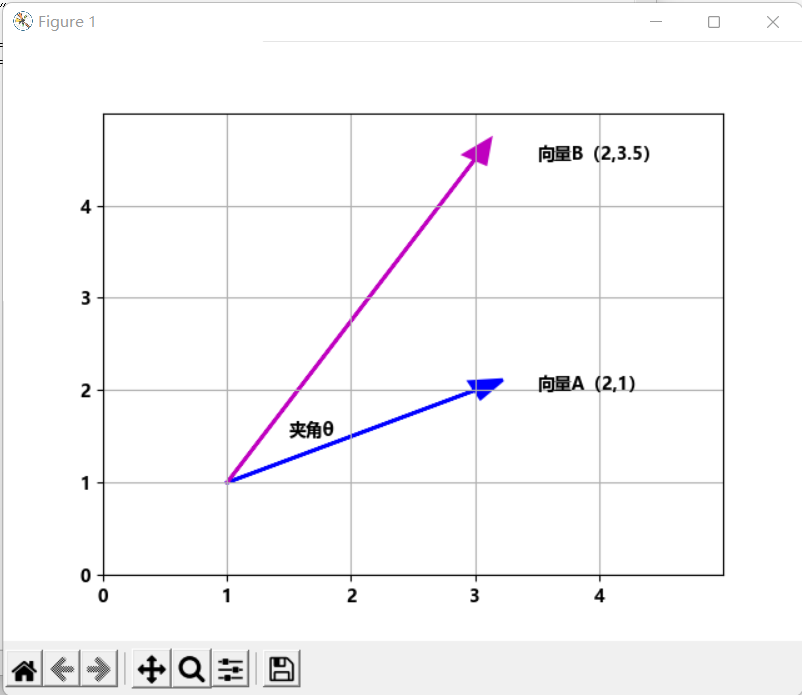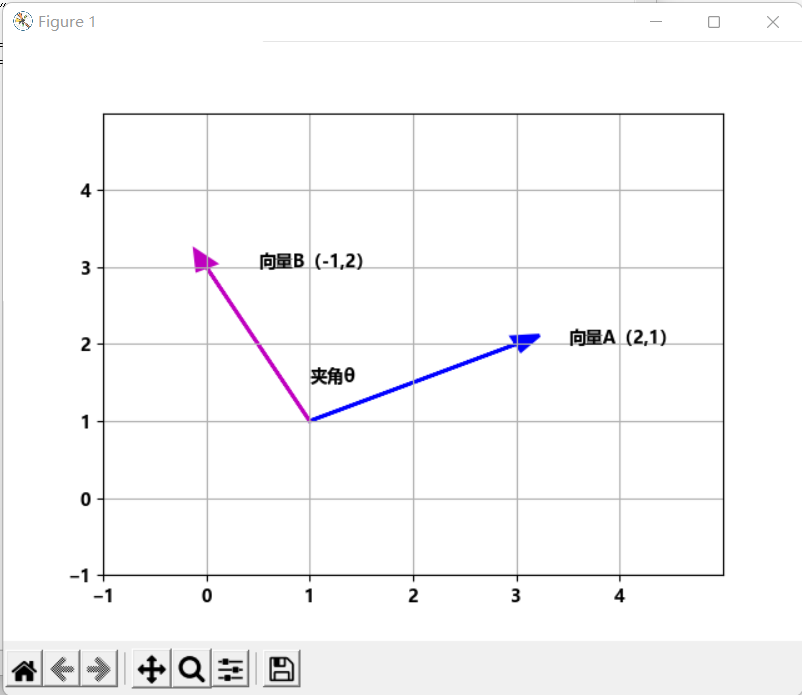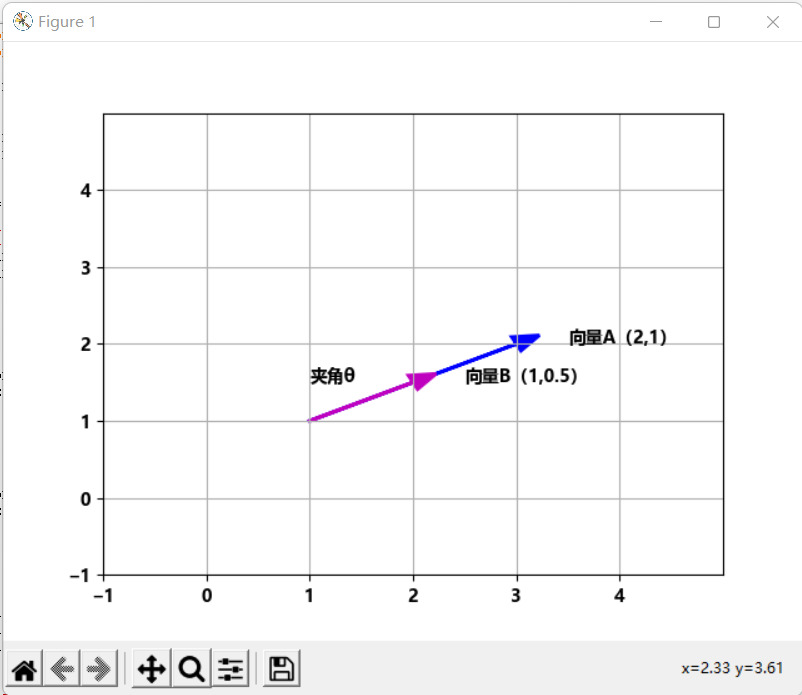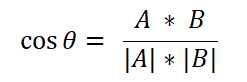2423字，阅读需时9分钟import numpy as np
import matplotlib.pyplot as plt

plt.rc("font",family='MicroSoft YaHei',weight="bold")

# 定义向量A和B
A = np.array([2,1])
B = np.array([2,3.5])

# 创建坐标轴
ax = plt.axes()

#  (1,1)为起点，绘制向量A和B

# 设置X轴范围
plt.xlim(0,5)

# 设置X轴刻度
major_xticks = np.arange(0, 5)
ax.set_xticks(major_xticks)

# 设置Y轴范围
plt.ylim(0, 5)

# 设置Y轴刻度
major_yticks = np.arange(0,5)
ax.set_yticks(major_yticks)

# 绘制网格线
plt.grid(b=True, which='major')

# 绘制标注
ax.text(A+1.5,A+1,"向量A（2,1）")
ax.text(B+1.5,B+1,"向量B（2,3.5）")
ax.text(1.5,1.5,"夹角θ")
# 显示图像
plt.show()【例1】 计算例句1和例句2的相似度

import  numpy as np
# 定义向量A和B
A = np.array([1,1,2,1,1,0,0])
B = np.array([0,1,2,1,0,1,1])

# 计算点积
dot = np.dot(A,B)
# 计算向量A的模长
ma = np.linalg.norm(A)
# 计算向量B的模长
mb = np.linalg.norm(B)
# 计算向量A和B的相似度
sim = dot/(ma*mb)
print('相似度为%.6f' % (sim))